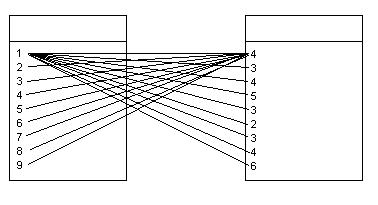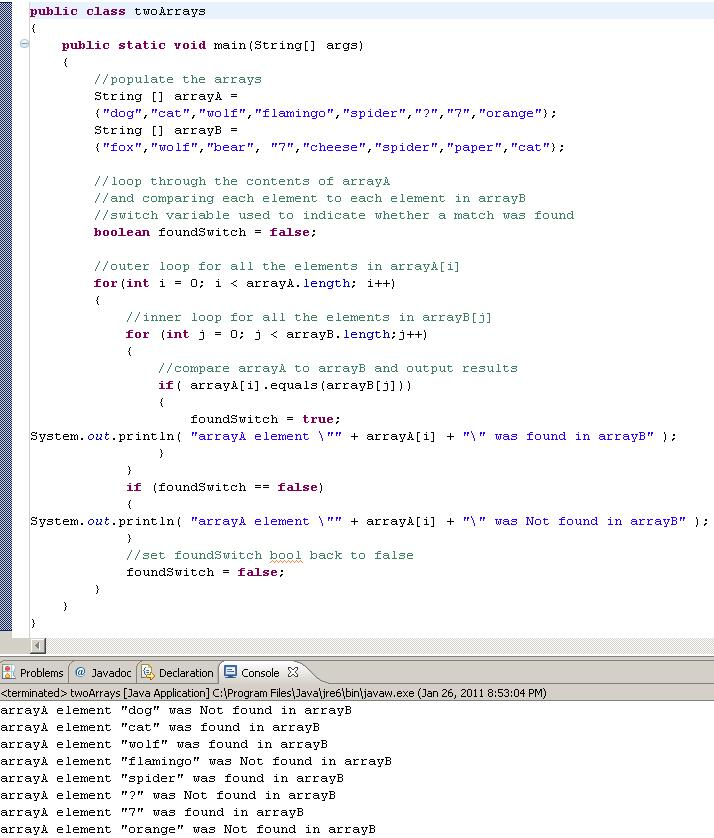# JAVA: How to compare two arrays

Compare the content/objects of two String Arrays. Loop through them comparing every element from one Array to another.## 11 thoughts on “JAVA: How to compare two arrays”

1.venu

Thanks, it helped me a lot

2.Hlaing Myo

Thank you so much, this help me a lot.

3.Joseph

How i can use compareto Method to get a new complete array for the above question:
String [] arrayA = {”cat”, “dog”,”wolf”};
String [] arrayB = {“fox”,”spider”,”paper”};
String[] arrayC = {arrayA.length+ arrayB.length}

you campare one by one to get array C using compareTo method.

thanxs

4.Joseph

public class Opdracht {

public static void main(String[] args) {

String [] A = {“Ali”,”Jareon”,”Sara”};
String [] B = {“Ceon”,”Rene”,”Peter”};
String [] C = new String[A.length + B.length];

int i ;
int k ;
int n ;

for(i = 0; i < A.length; i ++) {
for(n = 0; n < A.length && n < B.length ; n ++ ) {
for( k = 0; k < B.length; k++) {
System.out.println(A[i].compareToIgnoreCase(B[k]) + "");
}
}
}
}
}

what am i doing weong?

1.MantasCode Post author

I’m not sure what you’re trying to do here.

How i can use compareto Method to get a new complete array for the above question:
one by one to get array C using compareTo method.

What do you want this new complete array to be populated with? The results from the compareTo method?

5.michael

thanks for this , it help me a lot in my Java-Programming final Project. Thanks for the idea.

6.reyn

How can I compare if 1 is equal to 0? the input is in txt file (notepad)
example:
Input.txt
1100
100
101
10
————
Output:
Equal!
Not Equal!
Not Equal!
Equal

7.Humz

Thank you so much for this! So useful for my project.

However, I am having one issue… the console is outputting multiple lines for each result. On yours there is only one line per value, but mine is giving loads…

8.Indian

Thank You

9.Nuno

This post is exactly what I was looking for!
I needed to compare all members of 2 string arrays, but I didn’t know how to point out exclusive values found in one array only. That boolean does the trick, clever!